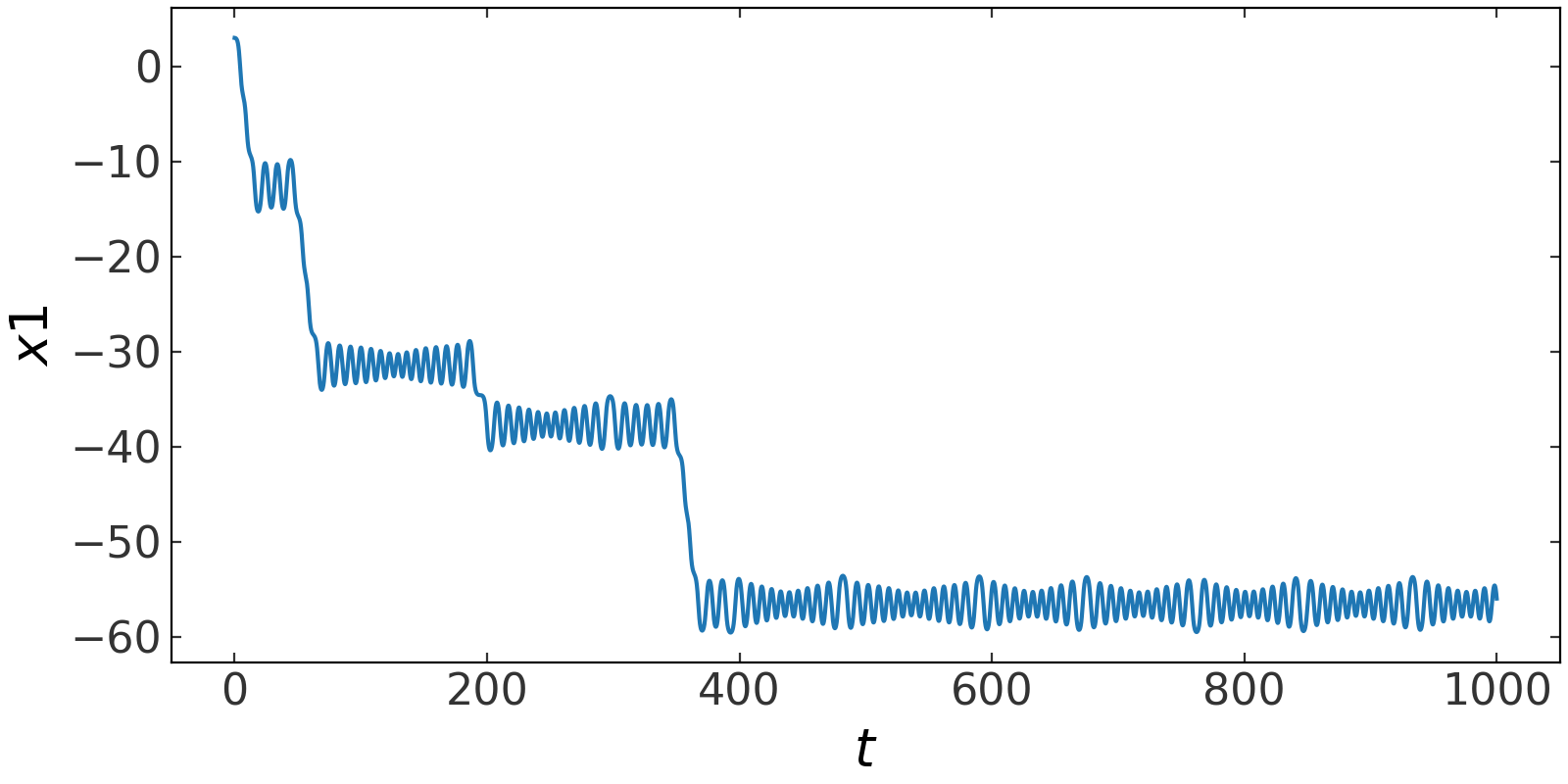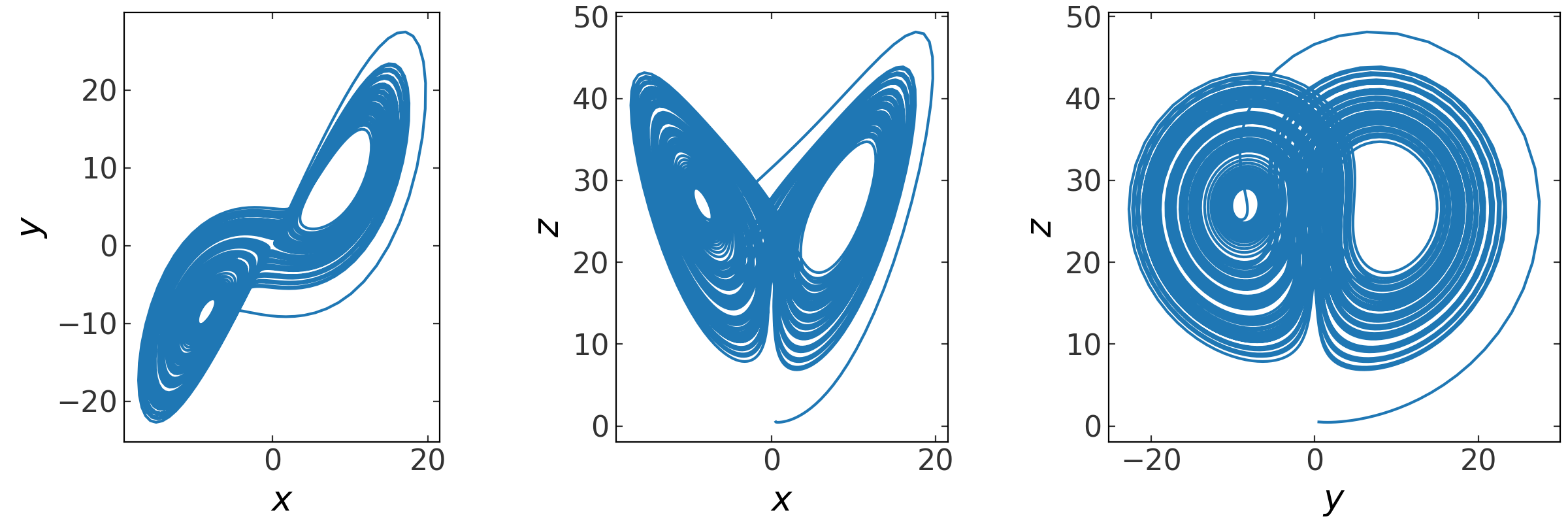# Integration (gala.integrate)¶

## Introduction¶

Scipy provides numerical ODE integration functions but they aren’t very user friendly or object oriented. This subpackage implements the Leapfrog integration scheme (not available in Scipy) and provides wrappers to higher order integration schemes such as a 5th order Runge-Kutta and the Dormand-Prince 85(3) method.

For code blocks below and any pages linked below, I assume the following imports have already been executed:

>>> import astropy.units as u
>>> import numpy as np
>>> import gala.integrate as gi
>>> from gala.units import galactic


## Getting Started¶

All of the integrator classes have the same basic call structure. To create an integrator object, you pass in a function that evaluates derivatives of, for example, phase-space coordinates, then you call the run method while specifying timestep information.

The integration function must accept, at minimum, two arguments: the current time, t, and the current position in phase-space, w. The time is a single floating-point number and the position will have shape (ndim, norbits) where ndim is the full dimensionality of the phase-space (e.g., 6 for a 3D coordinate system) and norbits is the number of orbits. These inputs will not have units associated with them (e.g., they are not astropy.units.Quantity objects). An example of such a function (that represents a simple harmonic oscillator) is:

>>> def F(t, w):
...     x,x_dot = w
...     return np.array([x_dot, -x])


Even though time does not explicitly enter into the equation, the function must still accept a time argument. We will now create an instance of LeapfrogIntegrator to integrate an orbit:

>>> integrator = gi.LeapfrogIntegrator(F)


To actually run the integrator, we need to specify a set of initial conditions. The simplest way to do this is to specify an array:

>>> w0 = np.array([1.,0.])


However this then causes the integrator to work without units. The orbit object returned by the integrator will then also have no associated units. For example, to integrate from these initial conditions with a time step of 0.5 for 100 steps:

>>> orbit = integrator.run(w0, dt=0.5, n_steps=100)
>>> orbit.t.unit
Unit(dimensionless)
>>> orbit.pos.xyz.unit
Unit(dimensionless)


We can instead specify the unit system that the function (F) expects, and then pass in a PhaseSpacePosition object with arbitrary units in as initial conditions:

>>> import gala.dynamics as gd
>>> from gala.units import UnitSystem
>>> usys = UnitSystem(u.m, u.s, u.kg, u.radian)
>>> integrator = gi.LeapfrogIntegrator(F, func_units=usys)
>>> w0 = gd.PhaseSpacePosition(pos=[100.]*u.cm, vel=*u.cm/u.yr)
>>> orbit = integrator.run(w0, dt=0.5, n_steps=100)
>>> orbit.t.unit
Unit("s")


Now the orbit object will have quantities in the specified unit system.

### Example: Forced pendulum¶

Here we demonstrate how to use the Dormand-Prince integrator to compute the orbit of a forced pendulum. We will use the variable q as the angle of the pendulum with respect to the vertical and p as the conjugate momentum. Our Hamiltonian is

$H(q,p) = \frac{1}{2}p^2 + \cos(q) + A\sin(\omega_D t)$

so that

$\begin{split}\dot{q} &= p\\ \dot{p} &= -\sin(q) + A\omega_D\cos(\omega_D t)\end{split}$

Our derivative function is then:

>>> def F(t, w, A, omega_D):
...     q,p = w
...     q_dot = p
...     p_dot = -np.sin(q) + A*omega_D*np.cos(omega_D*t)
...     return np.array([q_dot, p_dot])


This function has two arguments – $$A$$ (A), the amplitude of the forcing, and $$\omega_D$$ (omega_D), the driving frequency. We define an integrator object by specifying this function, along with values for the function arguments:

>>> integrator = gi.DOPRI853Integrator(F, func_args=(0.07, 0.75))


To integrate an orbit, we use the run method. We have to specify the initial conditions along with information about how long to integrate and with what stepsize. There are several options for how to specify the time step information. We could pre-generate an array of times and pass that in, or pass in an initial time, end time, and timestep. Or, we could simply pass in the number of steps to run for and a timestep. For this example, we will use the last option. See the API below under “Other Parameters” for more information.:

>>> orbit = integrator.run([3.,0.], dt=0.1, n_steps=10000)


We can plot the integrated (chaotic) orbit:

>>> fig = orbit.plot(subplots_kwargs=dict(figsize=(8,4)))### Example: Lorenz equations¶

Here’s another example of integrating the Lorenz equations, a 3D nonlinear system:

>>> def F(t,w,sigma,rho,beta):
...     x,y,z,px,py,pz = w
...     return np.array([sigma*(y-x), x*(rho-z)-y, x*y-beta*z, 0., 0., 0.]).reshape(w.shape)
>>> sigma, rho, beta = 10., 28., 8/3.
>>> integrator = gi.DOPRI853Integrator(F, func_args=(sigma, rho, beta))
>>> orbit = integrator.run([0.5,0.5,0.5,0,0,0], dt=1E-2, n_steps=1E4)
>>> fig = orbit.plot()## API¶

### gala.integrate Package¶

#### Functions¶

 parse_time_specification(units[, dt, …]) Return an array of times given a few combinations of kwargs that are accepted – see below.

#### Classes¶

 DOPRI853Integrator(func[, func_args, …]) This provides a wrapper around Scipy’s implementation of the Dormand-Prince 85(3) integration scheme. LeapfrogIntegrator(func[, func_args, …]) A symplectic, Leapfrog integrator. RK5Integrator(func[, func_args, func_units, …]) Initialize a 5th order Runge-Kutta integrator given a function for computing derivatives with respect to the independent variables.